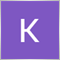Need help with"mamdanifuzzysystem"10

Hi!

I created a script to show my problem. To create the class works fine, the problem is with the result processing. The first iteration is ok, but if I wannt a second the, Inputs.Clear() dissapear the firstTerm object. To see more please run the script.

//+------------------------------------------------------------------+
//|                                                 Fuzzy tester.mq4 |
//|                        Copyright 2021, MetaQuotes Software Corp. |

//+------------------------------------------------------------------+
#property version   "1.00"
#property strict

#include <Math\Fuzzy\mamdanifuzzysystem.mqh>
CMamdaniFuzzyRule *rule1 ,*rule2, *rule3;

//+------------------------------------------------------------------+
//| Script program start function                                    |
//+------------------------------------------------------------------+
void OnStart()
{
CMamdaniFuzzySystem *OurFuzzy=new CMamdaniFuzzySystem();
CList *Inputs=new CList;

CFuzzyVariable *firstInput=  new CFuzzyVariable("diff", 0.0, 10.0);
CFuzzyVariable *fuzzyOut  = new CFuzzyVariable("out",0.0, 10.0);

CDictionary_Obj_Double *firstTerm= new CDictionary_Obj_Double;
CDictionary_Obj_Double *Output;

firstInput.Terms().Add(new CFuzzyTerm("sell",     new CZ_ShapedMembershipFunction   (3.0, 7.0)));
firstInput.Terms().Add(new CFuzzyTerm("neutral",  new CZ_ShapedMembershipFunction   (3.0, 10.0)));

fuzzyOut.Terms().Add(new CFuzzyTerm("neutral",    new CNormalMembershipFunction    (3.0, 7.0)));
fuzzyOut.Terms().Add(new CFuzzyTerm("sell",       new CS_ShapedMembershipFunction  (3.0, 10.0)));

rule2  = OurFuzzy.ParseRule("if (diff is sell)      then (out is sell)");
rule3  = OurFuzzy.ParseRule("if (diff is neutral)   then (out is neutral)");

for(int i=1; i<10; i++)
{
Print("Iteration: ", i);

//set i as the first input value
firstTerm.SetAll(firstInput,i);

Print("Iteration value: ",firstTerm.Value());

//clear previous inputs
Inputs.Clear(); //<the clear process delete the firstTerm object!

Print("Number of data on the input: ",Inputs.Total());

//calculate
CList *FuzzResult=OurFuzzy.Calculate(Inputs);

//get result
Output=FuzzResult.GetNodeAtIndex(0);
double res = Output.Value();

//print result
Print("Result: ",i,"  ",res);

//delete object
delete FuzzResult;
}

delete Inputs;
delete OurFuzzy;
}
//+------------------------------------------------------------------+